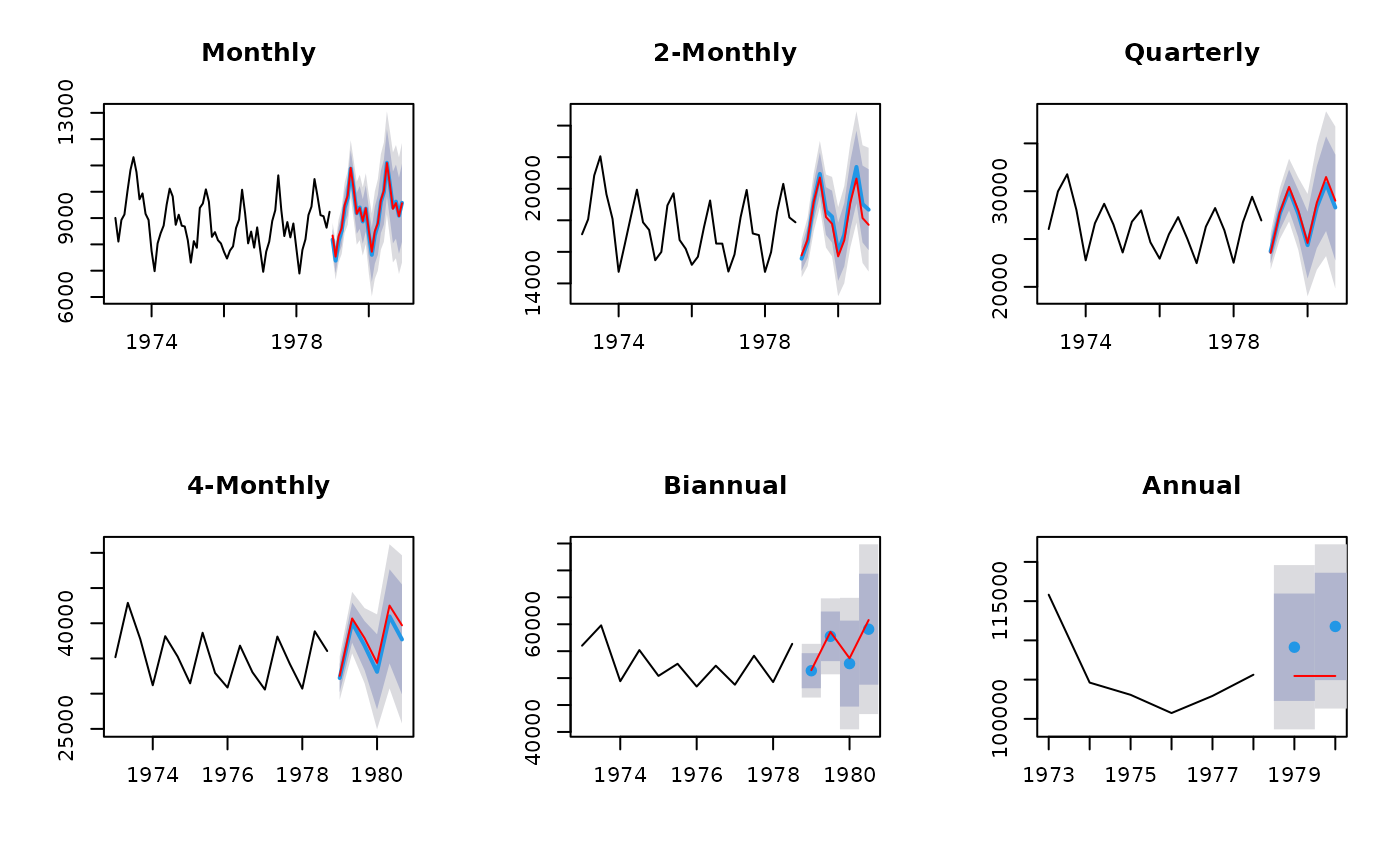Takes forecasts of time series at all levels of temporal aggregation and combines them using the temporal hierarchical approach of Athanasopoulos et al (2016).

reconcilethief(forecasts, comb = c("struc", "mse", "ols", "bu", "shr", "sam"),
mse = NULL, residuals = NULL, returnall = TRUE, aggregatelist = NULL)

Arguments

forecasts List of forecasts. Each element must be a time series of forecasts, or a forecast object. The number of forecasts should be equal to k times the seasonal period for each series, where k is the same across all series. Combination method of temporal hierarchies, taking one of the following values: "struc"Structural scaling - weights from temporal hierarchy "mse"Variance scaling - weights from in-sample MSE "ols"Unscaled OLS combination weights "bu"Bottom-up combination -- i.e., all aggregate forecasts are ignored. "shr"GLS using a shrinkage (to block diagonal) estimate of residuals "sam"GLS using sample covariance matrix of residuals A vector of one-step MSE values corresponding to each of the forecast series. List of residuals corresponding to each of the forecast models. Each element must be a time series of residuals. If forecast contains a list of forecast objects, then the residuals will be extracted automatically and this argument is not needed. However, it will be used if not NULL. If TRUE, a list of time series corresponding to the first argument is returned, but now reconciled. Otherwise, only the most disaggregated series is returned. (optional) User-selected list of forecast aggregates to consider

Value

List of reconciled forecasts in the same format as forecast. If returnall==FALSE, only the most disaggregated series is returned.